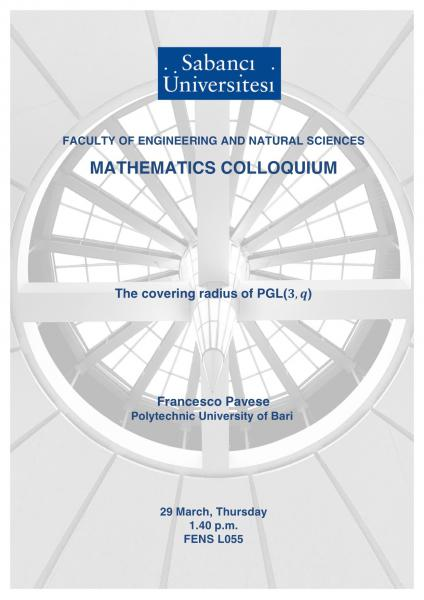## Mathematics Colloquium: The covering radius of PGL(3, q)

### Mathematics Colloquium: The covering radius of PGL(3, q)Speaker:  Francesco Pavese

Title: The covering radius of PGL(3, q)

Date/Time:  Thursday, 29 March @ 13:40 pm

Place: FENS - L055

You are cordially invited to attend the colloquium given by Francesco Pavese (Polytechnic University of Bari) on Thursday, 29 March 2018, in FENS-L055 at 1.40 pm.

Abstract:  Let Symn denote the symmetric group consisting of all permutations on n elements. For two permutations ρ,τ ∈ Symn the Hamming distance is given by d(ρ,τ) = |{x ∈ [1,2,...,n] | ρ(x) ̸= τ(x)}|. Equivalently d(ρ,τ) = n−|Fix(ρτ−1)|, where Fix(ρτ−1) denotes the number of fixed points by ρτ−1. The symmetric group Symn, equipped with the Hamming distance is a metric space. A permutation code C is a set of permutations of Symn. The codewords are the permutations and the code length is n. The minimum Hamming distance of the code C is the minimum d(ρ,τ) taken over all pairs ρ, τ of distinct permutations of C. The covering radius cr(C) of C is the smallest R such that the balls of radius R with centers at the elements of C cover the whole space. In this talk, with a completely geometric approach, a proof establishing the covering radius of PGL(3, q) ≤ Symq2+q+1 will be outlined.

Contact: Yasemin Şengül Tezel